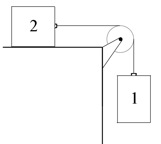8.01SC | Fall 2016 | Undergraduate

# Classical Mechanics

Week 10: Rotational Motion

## PS.10.1 Blocks and Massive Pulley

« Previous | Next »

A pulley of mass $$\displaystyle m_ p$$, radius $$\displaystyle R$$, and moment of inertia about its center of mass $$\displaystyle I_{cm}$$, is attached to the edge of a table. An inextensible string of negligible mass is wrapped around the pulley and attached on one end to block 1 that hangs over the edge of the table. The other end of the string is attached to block 2 which slides along a table. The coefficient of sliding friction between the table and the block 2 is $$\displaystyle \mu _ k$$. Block 1 has mass $$\displaystyle m_1$$ and block 2 has mass $$\displaystyle m_2$$, with $$\displaystyle m_1>\mu _ k m_2$$. At time $$\displaystyle t=0$$, the blocks are released from rest. At time $$\displaystyle t=t_1$$, block 1 hits the ground. Let $$\displaystyle g$$ denote the gravitational constant.Find the direction and magnitude of the acceleration of the block 1 hanging over the edge of the table. The positive direction is chosen to be downward.

« Previous | Next »